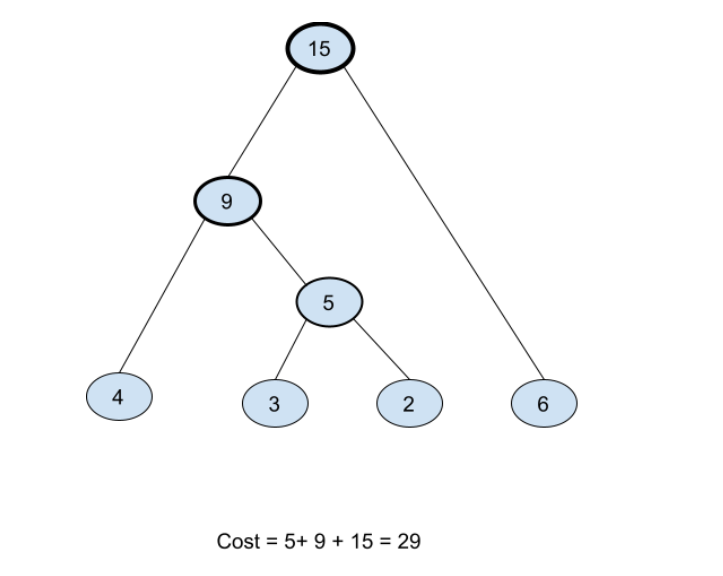# Connect N Ropes

## Introduction

The ‘Connect n ropes’ problem is one of the popular questions commonly asked in technical interviews. This problem is the implementation of Huffman coding, well known greedy approach used to find greedy-based solutions.

## Problem statement

There are n ropes of different lengths; connect n ropes into one rope. The cost for connecting two ropes is equal to the sum of their lengths. We need to connect all the ropes with minimum cost. Find the minimum cost.

## Example

Input : n=4

arr[] = {4,3,2,6}

Output : 29Here, we are extracting the minimum lengths from the list in every step to connect the ropes. To implement such action, we need min-heap as in min-heap smallest value always remains at the top(i.e., root).

In the above example, the 1st and 2nd smallest lengths are 2 and 3, respectively. So, we connect them first(2+3=5 ).

Now the available lengths are 4, 5, 6, where the 1st and 2nd smallest lengths are 4 and 5 respectively(4+5=9). After connecting them, the available lengths are 9, 6.

Connecting these two lengths, the final length of the connected ropes (9+6)=15

We are connecting the ropes 3 times to get the final length. So the cost is

5+9+15=29.

## Method

Here we follow the optimal merging pattern to minimize the cost of connecting the n ropes. We will always take the greedy approach of connecting 1st and 2nd smallest ropes and recur for the remaining ropes along with new connected rope.

Algo

1. Create a min-heap and insert all lengths of the ropes into the min-heap.
2. Do the following while the number of elements in the min-heap is greater than one.
1. Extract 1st and 2nd smallest lengths from min-heap
2. Add two extracted values and insert the added value(length of connected rope) to the min-heap.
3. need to maintain a variable for total cost and keep incrementing it by the sum of extracted values.
3. Return the value of this total cost.

Output

## Complexity analysis

In the ‘connect n ropes’ problem O(nlogn) is the time complexity as heap operation takes O(logn) time for inserting or extracting elements.

O(n) space complexity for the min-heap.

## FAQs

1. What is Huffman coding?
Huffman code is a particular type of optimal prefix code that is commonly used as a lossless data compression algorithm.

2. For which kind of problems we should apply the greedy-based approach?
The problems where choosing local optimal solutions leads to the global optimal solution, are the best fit for the greedy-based approach.

3. How to implement min-heap using C++ STL library?
In C++, STL library priority_queue is by default made max-heap. To use priority_queue as min-heap, we need to add two extra arguments as shown below.

priority_queue<type, vector<type>, greater<type> > pq;

## Key Takeaways

This article on the problem ‘connect n ropes’ discussed the approach of connecting n ropes with minimum cost using the concept of optimal merging pattern.

Apart from this, you can practice a wide range of coding questions commonly asked in interviews in CodeStudio. Along with coding questions, we can also find the interview experience of scholars working in renowned product-based companies here.

Happy learning!